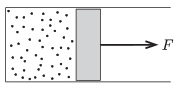Mathematical and Physical Journal
for High Schools
Issued by the MATFUND Foundation
 Already signed up? New to KöMaL?

#Problem P. 4610. (February 2014)

P. 4610. There is a sample of ideal gas in a cylinder shaped container. The symmetry axis of the cylinder is horizontal and at one end it is closed by frictionlessly moveable piston. Initially the volume of the gas is V1, its temperature is T1 and its pressure is p1, which is the same as the ambient (and later on as well constant) air pressure.The sample of gas is taken through the following processes n times: It is heated at constant pressure to a temperature of T2, and then it is cooled back to the temperature of T1 keeping its volume constant at its reached value. While the gas is heated at constant pressure an external constant force is exerted on the piston, which force is different at the different heating steps. During the whole process the total work done is W.

a) To what value will the volume of the gas increase after the n-th step?

b) What is the total work W done by the external force?

(5 pont)

Deadline expired on March 10, 2014.

Sorry, the solution is available only in Hungarian. Google translation

Megoldásvázlat. $\displaystyle a)$ $\displaystyle V_{n+1}=\left(\frac{T_2}{T_1}\right)^n\cdot V_1.$

$\displaystyle b)$ $\displaystyle W_{n}= \left[ \left(\frac{T_2}{T_1}\right)^n-1-n\left(\frac{T_2}{T_1}-1\right) \right]p_1V_1.$

### Statistics:

 55 students sent a solution. 5 points: Antalicz Balázs, Balogh Menyhért, Bereczki Zoltán, Berta Dénes, Blum Balázs, Büki Máté, Demeter Dániel, Di Giovanni Márk, Fehér Zsombor, Forrai Botond, Holczer András, Horicsányi Attila, Janzer Barnabás, Kácsor Szabolcs, Kaposvári Péter, Kenderes Anett, Koncz Imre, Kovács Péter Tamás, Marosvári Kristóf, Németh Flóra Boróka, Sal Kristóf, Sárvári Péter, Seress Dániel, Szász Norbert Csaba, Trócsányi Péter, Varju Ákos, Virágh Anna, Wiandt Péter. 4 points: Asztalos Bogdán, Bugár 123 Dávid, Csathó Botond, Dinev Georgi, Fekete Panna, Kasza Bence, Körei Réka Enikő. 3 points: 1 student. 2 points: 15 students. 1 point: 3 students. 0 point: 1 student.

Problems in Physics of KöMaL, February 2014